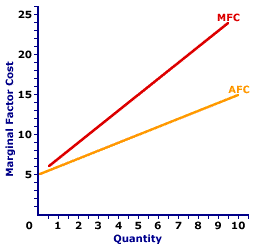Tuesday  August 9, 2022
 AmosWEB means Economics with a Touch of Whimsy!AGGREGATE EXPENDITURE EQUATION: An equation indicating that aggregate expenditures (AE) are the sum of consumption expenditures (C), investment expenditures (I), government purchases (G), and net exports (X-M), stated as: AE = C + I + G + (X-M). This equation surfaces in the Keynesian economic income-expenditure model in the form of the aggregate expenditures line. However, it's also central throughout the study of macroeconomics, including aggregate demand and the measurement of gross domestic product.MARGINAL FACTOR COST CURVE, MONOPSONY:

A curve that graphically represents the relation between marginal factor cost incurred by a monopsony for hiring an input and the quantity of input employed. A profit-maximizing monopsony hires the quantity of input found at the intersection of the marginal factor cost curve and marginal revenue product curve. The marginal factor cost curve for a monopsony with market control is positively sloped and lies above the average factor cost curve.
Monopsony is a market structure with a single buyer or in terms of factor markets, a single employer. This means that monopsony is a price maker with control over the buying side of the market. Market control means monopsony faces a positively-sloped supply curve. To buy a larger quantity, it must pay a higher price.

The marginal factor cost curve reflects the degree of market control held by a firm. For a perfectly competitive firm with no market control, the marginal factor cost curve is a horizontal line. For firms with more market control, especially monopsony, the marginal factor cost curve is positively sloped and lies above the average factor cost curve.

Marginal Factor Cost Curve,
MonopsonyThe marginal factor cost curve (MFC) for OmniKing Island Resort is displayed in the exhibit to the right. Key to this curve is that OmniKing is a monopsony buyer of workers and thus faces a positively-sloped supply curve. Larger quantities of input can be had only with higher prices.

The vertical axis measures marginal factor cost and the horizontal axis measures the quantity of input (workers). Although quantity on this particular graph stops at 10 workers, it could go higher.

This curve indicates that if OmniKing hires the first worker, then marginal factor cost is \$6. Alternatively, if it hires the tenth worker, then marginal factor cost is \$24.

For reference, the factor supply curve (and average factor cost curve, AFC) is also presented in the exhibit. The key observation is that the positively-sloped marginal factor cost curve (MFC) lies above the supply curve (AFC) facing OmniKing for hiring labor. The positioning of the marginal factor cost curve above the average factor cost curve reflects the mathematical relation between an average and a marginal.

Although this marginal factor cost curve is based on the employment activity of OmniKing Island Resort, a well-known monopsony firm, it works for any buyer with market control. Monopsonistic competition and oligopsony firms that also face positively-sloped supply curves generate comparable marginal factor cost curves.

 <= MARGINAL FACTOR COST CURVE MARGINAL FACTOR COST CURVE, PERFECT COMPETITION =>Recommended Citation:

MARGINAL FACTOR COST CURVE, MONOPSONY, AmosWEB Encyclonomic WEB*pedia, http://www.AmosWEB.com, AmosWEB LLC, 2000-2022. [Accessed: August 9, 2022].

Check Out These Related Terms...

Or For A Little Background...

And For Further Study...
Search Again?WHITE GULLIBON[What's This?] Today, you are likely to spend a great deal of time lost in your local discount super center trying to buy either a birthday gift for your mother or a weathervane with a horse on top. Be on the lookout for infected paper cuts.Your Complete ScopeOnly 1% of the U.S. population paid income taxes when the income tax was established in 1914."Work is an extension of personality. It is achievement. It is one of the ways in which a person defines himself, measures his worth ‚ and his humanity. "-- Peter Drucker, authorABEAssociation of Business ExecutivesA PEDestrian's Guide Xtra CreditTell us what you think about AmosWEB. Like what you see? Have suggestions for improvements? Let us know. Click the User Feedback link.| | | | | | | | | | |
| | | |

Thanks for visiting AmosWEB